### UH-OH V. 2.1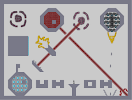Hover over the thumbnail for a full-size version.

Author [[N]] author:[[n]] n-art unrated 2006-10-27 4 more votes required for a rating. \$UH-OH V. 2.1#[[N]]#none#0000000000000000000000000000000111110000031140000000001111100003500240000000011111001Q1000010000000011111000010O0010000000011111000024Q035000000000000000000211500000000000000024000000000000000000000024001100003114000000000020001000350024000040000000110001000010002140000000000010010100002100000011000240035000000000000NP00002115000000000000011000000000000000000000000000000000000000000000400000000000000000000001110000000000000000000050000000000000000000000000000000000000000000011000000000000000000000PN000000000000000000000110000000000000000000000000003114000000000000011000350024000000000000NP00010000F0000000000001100010000G0000000000000000024003500000000000000000021150000000000000000000000000000000000000000|5^672,156!5^672,144!5^672,132!5^444,492!5^672,120!5^672,108!0^265,288!0^263,287!0^260,285!0^258,283!0^254,281!0^249,279!0^245,279!0^245,279!0^237,283!0^237,283!0^233,283!0^232,281!0^232,280!0^232,280!0^232,278!0^232,275!0^235,273!0^239,269!0^244,267!0^249,267!0^249,236!0^252,231!0^255,228!0^258,226!0^259,224!0^264,223!0^266,223!0^265,223!0^264,225!0^263,226!0^260,231!0^260,237!0^261,241!0^264,241!0^269,239!0^271,235!0^280,232!0^284,232!0^288,232!0^285,232!0^283,234!0^279,236!0^275,238!0^274,243!0^247,235!0^246,233!0^241,231!0^239,228!0^238,224!0^236,222!0^234,217!0^233,216!0^232,216!0^231,219!0^230,221!0^230,224!0^229,228!0^229,233!0^229,236!0^229,239!0^230,241!0^231,248!0^230,248!0^225,248!0^223,251!0^220,252!0^218,254!0^216,257!0^216,258!0^217,258!0^221,257!0^231,258!0^236,259!0^242,259!0^248,261!0^250,263!0^248,263!0^236,259!0^231,258!0^226,258!0^242,282!0^289,230!0^289,235!0^288,237!0^286,250!0^286,245!0^289,240!0^288,241!0^287,250!0^289,252!0^289,253!0^290,255!0^291,255!0^294,254!0^292,257!0^289,260!0^289,263!12^324,300!12^318,294!12^324,294!12^330,294!12^324,288!12^330,288!12^330,282!12^336,282!12^336,288!12^342,282!12^342,276!12^336,276!12^348,270!12^348,276!12^342,270!12^354,264!12^348,264!12^354,270!12^354,258!12^360,258!12^360,264!12^360,252!12^366,252!12^366,258!12^372,246!12^372,252!12^366,246!12^372,240!12^378,240!12^378,246!12^378,234!12^384,234!12^384,240!12^384,228!12^390,228!12^390,234!12^390,222!12^396,222!12^396,228!12^396,216!12^402,216!12^402,222!12^402,210!12^408,210!12^408,216!12^408,204!12^414,204!12^414,210!12^414,198!12^420,198!12^420,204!12^420,192!12^426,192!12^426,198!12^426,186!12^432,186!12^432,192!12^432,180!12^438,180!12^438,186!12^438,174!12^444,174!12^444,180!12^444,168!12^450,168!12^450,174!12^450,162!12^456,162!12^456,168!12^456,156!12^462,156!12^462,162!12^462,150!12^468,150!12^468,156!12^468,144!12^474,144!12^474,150!12^474,138!12^480,138!12^480,144!3^476,130!3^475,129!3^475,126!3^477,121!3^477,118!3^480,117!3^484,117!3^486,117!3^497,124!3^501,127!3^501,129!3^501,131!3^499,136!3^497,140!3^494,142!3^487,144!3^485,143!3^485,139!3^486,132!3^488,131!3^489,128!3^487,127!3^495,117!3^493,117!3^492,116!3^499,116!3^500,116!3^504,124!3^498,137!3^502,135!3^503,127!3^504,124!3^504,119!3^504,116!3^501,112!3^498,109!3^495,107!3^492,107!3^488,107!3^484,108!3^479,109!3^475,111!3^473,119!3^473,126!3^473,129!3^473,76!3^471,76!3^468,77!3^464,78!3^462,79!3^457,81!3^457,81!3^453,88!3^450,91!3^447,99!3^446,100!3^446,102!3^446,106!3^445,109!3^445,112!3^502,76!3^506,77!3^508,78!3^510,79!3^512,81!3^517,84!3^518,86!3^522,90!3^524,93!3^525,97!3^525,99!3^527,104!3^527,106!3^529,110!3^529,113!3^529,116!3^528,146!3^528,146!3^527,149!3^525,152!3^523,156!3^520,159!3^518,162!3^516,165!3^511,167!3^507,170!3^505,171!3^445,145!3^445,147!3^445,150!3^448,154!3^463,169!3^464,170!3^470,173!3^470,174!3^472,174!3^476,175!3^462,79!3^456,81!3^455,82!3^453,89!3^449,95!3^445,148!3^447,152!3^464,171!3^469,176!3^524,152!3^526,146!3^520,158!3^512,165!3^507,169!12^294,318!12^294,324!12^300,324!12^288,324!12^288,330!12^294,330!12^282,330!12^282,336!12^288,336!12^282,336!12^276,336!12^276,342!12^282,342!12^270,342!12^270,348!12^276,348!12^264,348!12^264,354!12^270,354!12^264,360!12^258,354!12^258,360!12^252,360!12^252,366!12^258,366!12^252,372!12^246,372!12^246,366!12^240,372!12^240,378!12^246,378!12^234,378!12^234,384!12^240,384!12^228,384!12^228,390!12^234,390!12^228,396!12^222,390!6^636,84,2,0,2,0!6^660,84,2,0,2,0!6^684,84,2,0,2,0!6^708,84,2,0,2,0!6^708,108,2,0,2,0!6^684,108,2,0,2,0!6^660,108,2,0,2,0!6^636,108,2,0,2,0!6^636,132,2,0,2,0!6^660,132,2,0,2,0!6^684,132,2,0,2,0!6^708,132,2,0,2,0!6^708,156,2,0,2,0!6^684,156,2,0,2,0!6^660,156,2,0,2,0!6^636,156,2,0,2,0!6^660,180,2,0,2,0!6^684,180,2,0,2,0!5^666,114!5^666,120!5^666,126!5^666,132!5^666,138!5^666,144!5^666,150!5^666,156!5^672,156!5^678,156!5^678,150!5^678,144!5^678,138!5^678,126!5^678,120!5^678,132!5^678,114!5^678,108!5^678,102!5^672,102!5^666,102!5^666,102!5^666,108!0^648,194!0^648,195!0^648,197!0^648,199!0^648,201!0^649,204!0^649,205!0^650,208!0^652,211!0^652,212!0^652,215!0^653,217!0^655,220!0^655,222!0^656,224!0^658,228!0^659,231!0^659,234!0^662,237!0^665,244!0^667,250!0^668,251!0^696,191!0^695,193!0^695,199!0^695,200!0^694,202!0^693,203!0^692,205!0^692,206!0^692,209!0^692,210!0^691,212!0^690,214!0^689,216!0^687,218!0^685,219!0^684,222!0^684,223!0^684,226!0^682,229!0^682,231!0^682,233!0^681,236!0^681,239!0^679,241!0^675,243!0^675,245!0^675,249!0^675,250!0^674,252!0^671,257!0^663,218!0^672,218!0^680,218!0^665,227!0^675,227!0^668,235!0^673,236!0^671,239!0^671,245!4^648,90,1!4^672,90,1!4^696,90,1!4^696,114,1!4^720,114,1!4^648,114,1!4^624,114,1!4^624,138,1!4^648,138,1!4^696,138,1!4^720,138,1!4^696,162,1!4^648,162,1!4^672,186,1!5^648,84!5^672,84!5^696,84!5^696,108!5^648,108!5^624,108!5^624,132!5^648,132!5^696,132!5^720,132!5^696,156!5^648,156!5^672,180!12^288,72!12^300,72!12^312,72!12^324,72!12^336,72!12^336,84!12^348,84!12^360,96!12^348,96!12^336,96!12^324,96!12^312,96!12^300,96!12^288,96!12^276,96!12^264,96!12^276,84!12^288,84!12^300,84!12^312,84!12^324,84!12^324,108!12^312,108!12^300,108!12^288,108!12^276,108!12^264,108!12^264,120!12^264,132!12^276,132!12^276,120!12^288,120!12^288,132!12^288,144!12^276,144!12^264,144!12^276,156!12^288,168!12^288,168!12^288,156!12^300,168!12^300,156!12^312,156!12^312,168!12^324,168!12^324,156!12^336,168!12^336,156!12^348,156!12^348,144!12^348,132!12^360,132!12^360,120!12^348,120!12^360,108!12^348,108!12^336,108!12^336,120!12^312,120!12^300,120!12^300,132!12^312,132!12^300,144!12^312,144!12^324,144!12^336,144!12^324,120!12^336,132!12^360,144!12^354,168!12^354,162!12^360,162!12^360,168!12^366,168!12^366,174!12^360,174!12^372,174!12^372,180!12^366,180!12^372,186!12^378,186!12^378,180!12^384,186!12^384,192!12^378,192!12^390,192!12^390,198!12^384,198!12^396,198!12^390,204!12^420,222!12^414,228!12^420,228!12^426,228!12^426,234!12^420,234!12^426,240!12^432,234!12^432,240!8^162,228,0!8^162,240,0!8^162,252,0!8^162,264,0!8^162,276,0!8^162,288,0!8^162,300,0!8^162,312,0!8^162,324,0!12^438,240!12^438,246!12^432,246!12^438,252!12^444,252!12^444,246!12^450,252!12^450,258!12^444,258!12^450,264!12^456,264!12^456,264!12^456,264!12^456,258!12^462,264!12^462,270!12^456,270!12^462,276!12^468,276!12^468,270!12^468,282!12^474,282!12^474,276!12^480,282!12^480,288!12^474,288!12^480,294!12^486,294!12^486,288!12^486,300!12^492,300!12^492,294!12^492,306!12^498,306!12^498,300!12^498,312!12^504,312!12^504,306!12^510,312!12^510,318!12^504,318!12^510,324!12^516,324!12^516,318!12^522,324!12^522,330!12^516,330!12^522,336!12^528,336!12^528,330!12^534,336!12^534,342!12^528,342!12^534,348!12^540,348!12^540,342!12^546,348!12^546,354!12^540,354!12^546,360!12^552,360!12^552,354!12^558,360!12^558,366!12^552,366!12^564,366!12^564,372!12^558,372!12^570,372!12^570,378!12^564,378!12^576,378!12^576,384!12^570,384!12^582,384!12^582,390!12^570,384!12^576,390!12^588,390!12^588,396!12^582,396!12^594,396!12^594,402!12^588,402!12^600,402!12^600,408!12^594,408!12^606,408!12^600,414!12^606,414!12^612,414!12^612,420!12^606,420!12^618,420!12^618,426!12^612,426!12^624,426!12^624,432!12^618,432!12^630,432!12^630,432!12^630,432!12^630,438!12^624,438!12^636,438!12^636,444!12^630,444!12^642,444!12^642,450!12^636,450!12^648,450!12^648,456!12^642,456!12^654,456!12^654,462!12^648,462!2^666,324,1,0!2^660,396,1,0!2^660,564,1,0!2^636,570,0.707106781186547,-0.707106781186547!2^630,558,0.707106781186547,-0.707106781186547!2^624,546,0.707106781186547,-0.707106781186547!2^684,570,-0.707106781186547,-0.707106781186547!2^690,558,-0.707106781186547,-0.707106781186547!2^696,546,-0.707106781186547,-0.707106781186547!12^660,462!12^660,468!12^654,468!12^666,468!12^666,474!12^660,474!12^672,474!12^672,480!12^666,480!12^678,480!12^678,486!12^672,486!12^684,486!12^684,492!12^678,492!12^690,492!12^690,498!12^690,498!12^684,498!12^696,498!12^696,504!12^690,504!12^702,504!12^702,510!12^696,510!12^708,510!12^708,516!12^702,516!12^714,516!12^714,522!12^708,522!12^720,522!12^720,528!12^714,528!12^726,528!12^726,534!12^720,534!12^732,534!12^732,540!12^726,540!12^738,540!12^738,546!12^732,546!12^744,546!12^744,552!12^738,552!12^750,552!12^750,558!12^744,558!12^756,558!12^756,564!12^750,564!12^762,564!12^762,570!12^756,570!12^762,576!12^768,570!5^672,108!5^672,120!5^672,132!5^672,144!12^727,577!12^726,575!12^725,573!12^724,572!12^721,570!12^720,567!12^720,566!12^722,566!12^726,564!12^728,562!12^728,560!12^727,558!12^726,555!12^723,552!12^722,549!12^722,548!12^723,546!12^743,542!12^742,541!12^743,539!12^745,537!12^747,534!12^750,534!12^754,534!12^754,534!12^758,536!12^758,538!12^758,540!12^758,541!12^761,544!12^764,547!12^765,547!12^767,547!2^211,377,1,0!2^238,408,0,-1!2^200,368,1,0!2^244,419,0,-1!12^294,72!12^306,72!12^318,72!12^330,72!12^342,78!12^330,78!12^336,78!12^324,78!12^312,78!12^318,78!12^306,78!12^300,78!12^294,78!12^282,78!12^288,78!12^282,84!12^294,84!12^306,84!12^318,84!12^330,84!12^342,84!12^342,90!12^348,90!12^354,90!12^354,96!12^348,102!12^354,102!12^360,102!12^354,108!12^354,108!12^354,114!12^354,114!12^354,114!12^354,114!12^354,120!12^354,120!12^354,126!12^354,132!12^354,138!12^354,144!12^360,138!12^360,126!12^360,114!12^348,114!12^342,114!12^342,108!12^342,102!12^342,96!12^342,120!12^342,126!12^342,132!12^336,138!12^342,138!12^342,144!12^342,150!12^342,156!12^354,150!12^348,150!12^348,138!12^348,126!12^336,126!12^336,114!12^336,102!12^336,90!12^330,90!12^318,90!12^318,90!12^270,90!12^276,90!12^288,90!12^282,90!12^294,90!12^300,90!12^306,90!12^312,90!12^324,90!12^330,96!12^330,102!12^330,108!12^330,114!12^330,120!12^324,102!12^318,102!12^318,96!12^318,114!12^318,108!12^318,120!12^324,114!12^312,114!12^306,114!12^306,108!12^306,102!12^306,96!12^312,102!12^300,102!12^294,102!12^294,96!12^288,102!12^282,102!12^276,96!12^270,96!12^270,102!12^282,96!12^264,102!12^276,102!12^270,114!12^270,114!12^270,108!12^264,114!12^276,114!12^282,114!12^282,108!12^294,108!12^288,114!12^294,114!12^300,114!12^336,150!12^342,162!12^336,162!12^330,162!12^330,168!12^330,156!12^330,150!12^330,144!12^318,144!12^318,150!12^324,150!12^312,138!12^312,126!12^306,126!12^306,120!12^306,132!12^306,144!12^306,144!12^306,138!12^306,150!12^306,156!12^306,162!12^312,150!12^318,156!12^312,162!12^318,162!12^324,162!12^318,168!12^306,168!12^294,168!12^294,162!12^294,156!12^294,144!12^294,138!12^294,132!12^294,126!12^294,120!12^300,126!12^300,138!12^300,150!12^300,162!12^288,162!12^294,150!12^288,150!12^288,138!12^288,126!12^282,126!12^282,120!12^282,132!12^282,138!12^282,138!12^282,144!12^282,150!12^282,156!12^282,162!12^270,120!12^270,126!12^264,126!12^276,126!12^276,138!12^276,150!12^270,150!12^270,144!12^270,132!12^270,138!12^264,138!6^84,468,2,0,0,0!6^108,468,2,0,0,0!6^132,468,2,0,0,0!6^156,468,2,0,0,0!6^84,492,2,0,0,0!6^108,492,2,0,0,0!6^132,492,2,0,0,0!6^156,492,2,0,0,0!6^156,516,2,0,0,0!6^132,516,2,0,0,0!6^108,516,2,0,0,0!6^84,516,2,0,0,0!6^84,540,2,0,0,0!6^108,540,2,0,0,0!6^132,540,2,0,0,0!6^156,540,2,0,0,0!11^120,576,144,552!11^120,576,120,552!11^120,576,96,552!11^120,576,96,528!11^120,576,120,528!11^120,576,72,528!11^120,576,144,528!11^120,576,168,528!11^120,576,168,504!11^120,576,144,504!11^120,576,120,504!11^120,576,96,504!11^120,576,72,504!11^120,576,72,480!11^120,576,96,480!11^120,576,120,480!11^120,576,168,480!11^120,576,144,480!11^120,576,96,456!11^120,576,120,456!11^120,576,144,456!10^120,71!10^122,71!10^126,71!10^130,72!10^133,73!10^134,73!10^134,74!10^135,74!10^138,75!10^139,78!10^140,79!10^143,82!10^146,86!10^146,86!10^149,89!10^149,89!10^150,92!10^152,95!10^152,97!10^152,99!10^153,100!10^153,103!10^153,105!10^99,72!10^98,72!10^95,72!10^90,72!10^87,73!10^84,73!10^81,74!10^75,78!10^72,84!10^71,86!10^67,93!10^66,99!10^66,100!10^66,102!10^66,103!10^66,102!10^68,92!10^68,86!10^73,78!10^65,127!10^65,127!10^65,129!10^65,132!10^65,135!10^66,137!10^68,140!10^69,143!10^72,145!10^74,147!10^77,148!10^80,151!10^81,152!10^83,153!10^86,154!10^88,154!10^94,158!10^98,158!10^102,158!10^153,122!10^153,122!10^153,122!10^153,127!10^153,127!10^152,129!10^152,131!10^151,133!10^150,137!10^150,141!10^149,143!10^147,146!10^146,150!10^144,152!10^141,154!10^135,156!10^135,156!10^132,156!10^130,157!10^121,100!10^121,100!10^117,99!10^114,98!10^111,96!10^102,95!10^99,95!10^97,96!10^94,98!10^94,105!10^94,110!10^94,117!10^96,124!10^98,127!10^101,127!10^105,127!10^108,127!10^114,127!10^117,123!10^123,116!10^122,102!10^102,93!10^97,100!10^96,108!10^101,118!10^108,124!10^116,122!10^116,111!10^108,106!10^104,107!10^106,110!10^119,109!10^124,105!10^126,106!10^124,121!10^126,117!10^126,114!10^121,121!10^119,125!10^108,116!12^768,576# Please remember this is N Art and you do not play it (karan_and_kishan)! Fixed up the rocket drone quite a bit. Also added color to the zap drone thanks to tom_da_bomb (whos map is now listed in the credits). .:CREDITS:. -------------------------------------------------------- http://numa.notdot.net/map/63981 http://numa.notdot.net/map/64137 http://numa.notdot.net/map/63994 *NEW* http://numa.notdot.net/map/62946 -------------------------------------------------------- Thanks everyone for inspiring me. Rates and comments appreciated.

## Other maps by this author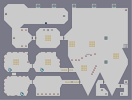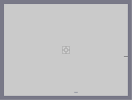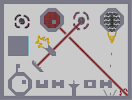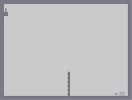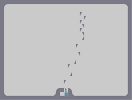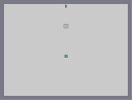Optional Funny Death #10 Uh-Oh Simply Annoying N Reaches High Speeds! Funny Death #11